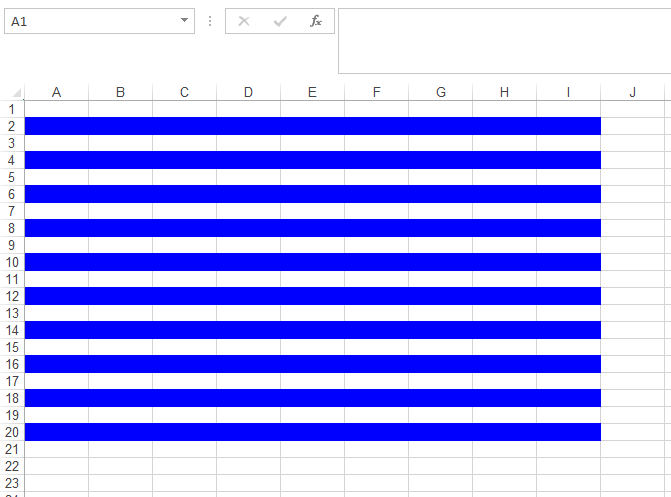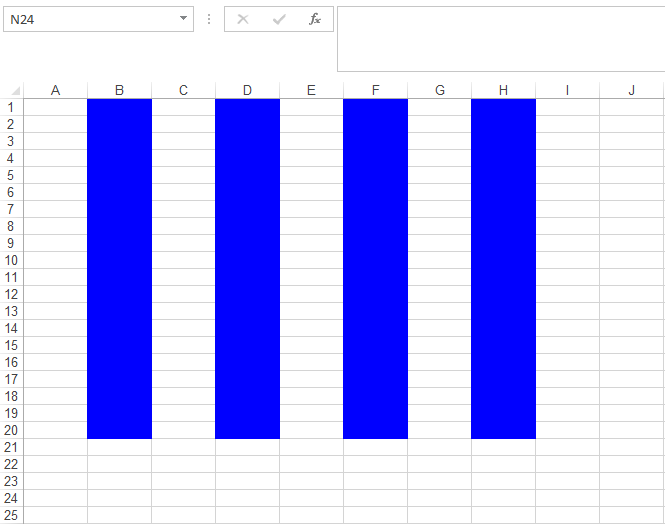# Apply Shading to Alternate Rows and Columns with Conditional Formatting

Contents
[ ]

## Apply Shading to Alternate Rows & Columns using Conditional Formatting

This article makes use of Excel’s built-in functions such as ROW, COLUMN & MOD. Here are little details of these functions for a better understanding of the code snippet provided ahead.

• ROW() function returns the row number of a cell reference. If the reference is omitted, it assumes that the reference is the cell address in which the ROW function has been entered in.
• COLUMN() function returns the column number of a cell reference. If the reference is omitted, it assumes that the reference is the cell address in which the COLUMN function has been entered in.
• MOD() function returns the remainder after a number is divided by a divisor, where the first parameter to the function is the numeric value whose remainder you wish to find and the second parameter is the number used to divide into the number parameter. If the divisor is 0, then it will return the #DIV/0! error.

Let us start writing some code to accomplish the goal with the help of Aspose.Cells for Java API.